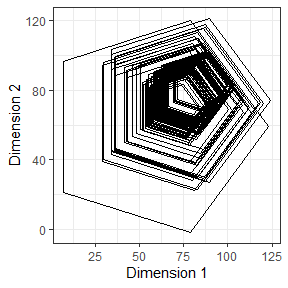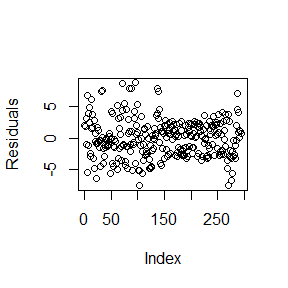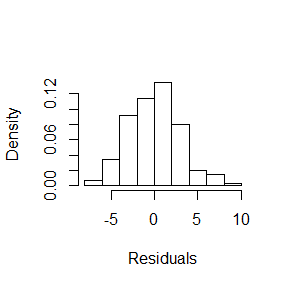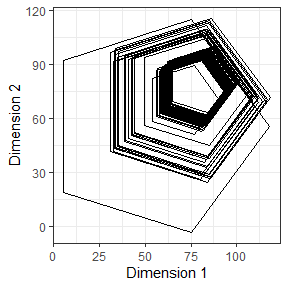# introdution-psda

#### November 28, 2019

library(psda)
#>
#> Attaching package: 'psda'
#> The following object is masked from 'package:stats':
#>
#>     na.omit

This vignette document is a brief tutorial for psda 1.3.2. Descriptive, auxiliary a modeling functions are presented and applied an example.

Data Science is fundamental to handle and extract knowledge about the data. Silva et al.  presented the Symbolic Polygonal Data Analysis as an approach to this task. The psda package is a toolbox to transform number in knowledge. We highlight some important characteristics of the package:

• It constructs symbolic polygonal data from classical data;
• It calculates symbolic polygonal descriptive measures;
• It models symbolic polygonal data through a symbolic polygonal linear regression model.

# WNBA 2014 Data

Women national basketball american (WNBA) dataset is used to demostrate the funcionality of the package. It has classical data with dimension 4022 by 6.

library(psda)
library(ggplot2)
data(wnba2014)
dta <- wnba2014

To construct the symbolic polygonal variables we need to have a class, i.e. a categorical variable. Then, we use the player_id variable

dta$player_id <- factor(dta$player_id)
head(dta)
#>   player_id team_pts opp_pts minutes fgatt efficiency
#> 1         1       89      77      36    21         35
#> 2         1       90      87      43    24         35
#> 3         1       94      93      35    21         43
#> 4         1       87      82      31    19         26
#> 5         1       75      72      35    14         16
#> 6         1       88      72      34    18         30

Next, we can obtain the center and radius of the polygon through the paggreg function. The only argument necessary is a dataset which has the first column as a factor (the class). From head function we can show the first six symbolic polygonal individuals in center and radius representation.

center_radius <- paggreg(dta)
head(center_radius$center, 6) #> team_pts opp_pts minutes fgatt efficiency #> 1 81.64706 77.17647 34.73529 18.02941 25.76471 #> 2 81.17647 83.26471 35.05882 16.08824 16.52941 #> 3 78.43333 77.80000 33.13333 15.36667 22.43333 #> 4 80.70968 78.09677 30.96774 15.83871 16.22581 #> 5 72.14706 75.20588 32.58824 15.02941 19.58824 #> 6 83.57576 73.45455 30.78788 11.42424 17.15152 head(center_radius$radius, 6)
#>   team_pts  opp_pts   minutes     fgatt efficiency
#> 1 20.14422 21.19732  7.774220  8.406135   18.96765
#> 2 21.88383 22.24551  6.803585  6.331597   15.54844
#> 3 17.89381 26.75766 10.180893  8.460592   15.42665
#> 4 20.67007 18.67158 12.727753 10.419172   17.41807
#> 5 21.85424 20.19168 12.081718  9.890127   18.52839
#> 6 16.82283 17.86121 10.121235  8.718232   14.96764

To construct the polygons it is necessary define the number of sides disered. We use as an example a pentagon, i.e. polygons with five vertices. The construction of polygons is given by psymbolic function that need of an object of the class paggregated and the number of vertices. To exemplify, we use the head function to show the first three individuals of the team_pts polygonal variable.

v <- 5
polygonal_variables <- psymbolic(center_radius, v)
head(polygonal_variables$team_pts, 3) #> [] #> [,1] [,2] #> [1,] 87.87197 100.80535 #> [2,] 65.35004 93.48754 #> [3,] 65.35004 69.80658 #> [4,] 87.87197 62.48877 #> [5,] 101.79128 81.64706 #> #> [] #> [,1] [,2] #> [1,] 87.93895 101.98923 #> [2,] 63.47208 94.03946 #> [3,] 63.47208 68.31348 #> [4,] 87.93895 60.36371 #> [5,] 103.06030 81.17647 #> #> [] #> [,1] [,2] #> [1,] 83.96283 95.45136 #> [2,] 63.95694 88.95105 #> [3,] 63.95694 67.91561 #> [4,] 83.96283 61.41531 #> [5,] 96.32715 78.43333 # Descriptive Measures After to obtain the symbolic polygonal data we can start to extract knowledge of this type of data through polygonal descriptive measure. Some of this measures are bi-dimensionals, because indicate the relation with the dimensions of the polygons . In this vignette we present the mean, variance, covariance and correlation as can be seen below: ## symbolic polygonal mean pmean(polygonal_variables$team_pts)
#>  75.7991 75.7991
pmean(polygonal_variables$opp_pts) #>  74.68993 74.68993 ## symbolic polygonal variance pvar(polygonal_variables$team_pts)
#>  126.1975 126.1975
pvar(polygonal_variables$opp_pts) #>  364.7798 364.7798 ## symbolic polygonal covariance pcov(polygonal_variables$team_pts)
#>  -2.379541
pcov(polygonal_variables$opp_pts) #>  -1.632418 ## symbolic polygonal correlation pcorr(polygonal_variables$team_pts)
#>  -0.01885568
pcorr(polygonal_variables$opp_pts) #>  -0.004475078 The construction of symbolic polygonal scatterplot is done through ggplot2 package, including all modification. From pplot we use a symbolic polygonal variable to plot the scatterplot. The graphic is a powerful tool to understand the data, for example, in this case, we can observe a pentagon with a radius greater than all. This can indicate outliers. # Visualization pplot(polygonal_variables$team_pts) + labs(x = 'Dimension 1', y = 'Dimension 2') +
theme_bw()# Modeling

To explain the behavior of a team_pts polygonal variable across fgaat, minutes, efficiency and opp_ptspolygonal variable, we use the polygonal linear regression model plr. The function needs of a formula and an environment containing the symbolic polygonal variables.

fit <- plr(team_pts ~ fgatt + minutes + efficiency + opp_pts, data = polygonal_variables)

The summary function is a method of plr. A summary of the polygonal linear regression model is showed from this method. In details, we can observe the quartile of the residuals, estimates of the parameters and its standard deviation. Besides, the statistical of test and the p-value is displayed.

s <- summary(fit)
s
#>
#> Call:
#> plr(formula = team_pts ~ fgatt + minutes + efficiency + opp_pts,
#>     data = polygonal_variables)
#>
#> Residuals:
#>    Min     1Q Median     3Q    Max
#> -7.525 -2.145  0.018  1.958  8.756
#>
#> Coefficients:
#>                      Estimate Std. Error z value  Pr(>|z|)
#> (center-intercept) 48.3670418  2.6609779 18.1764 < 2.2e-16 ***
#> center-fgatt       -0.1368189  0.1653467 -0.8275  0.408670
#> center-minutes     -0.0582787  0.0776782 -0.7503  0.453723
#> center-efficiency   0.3080644  0.1048899  2.9370  0.003586 **
#> center-opp_pts      0.3612354  0.0372591  9.6952 < 2.2e-16 ***
#> (radius-intercept) 14.0791683  1.0914791 12.8992 < 2.2e-16 ***
#> radius-fgatt       -0.0761514  0.2339476 -0.3255  0.745039
#> radius-minutes      0.0210633  0.0819685  0.2570  0.797390
#> radius-efficiency  -0.0042650  0.1075372 -0.0397  0.968392
#> radius-opp_pts      0.2777421  0.0094771 29.3066 < 2.2e-16 ***
#> ---
#> Signif. codes:  0 '***' 0.001 '**' 0.01 '*' 0.05 '.' 0.1 ' ' 1

We plot the residuals of the model from plot and the histogram.

plot(fit$residuals, ylab = 'Residuals')hist(fit$residuals, xlab = 'Residuals', prob = T, main = '')The fitted values to the model can be accessed from fitted method. The arguments are: (i) model that is an object of the class plr; (ii) a boolean, named polygon, if TRUE the output is the predicted polygons, otherwise, a vector with dimension 2n x 1 is computed, the first n individuals indicate the fitted center and the last the radius; (iii) vertices should be the number of vertices of the polygon selected previously. Besides, we print the first three fitted polygons and plot all from pplot.

fitted_polygons <- fitted(fit, polygon = T, vertices = v)
head(fitted_polygons, 3)
#> []
#>          [,1]     [,2]
#> [1,] 85.68981 98.15132
#> [2,] 63.98958 91.10049
#> [3,] 63.98958 68.28353
#> [4,] 85.68981 61.23270
#> [5,] 99.10128 79.69201
#>
#> []
#>          [,1]     [,2]
#> [1,] 85.42773 98.17383
#> [2,] 63.23195 90.96198
#> [3,] 63.23195 67.62395
#> [4,] 85.42773 60.41210
#> [5,] 99.14548 79.29297
#>
#> []
#>           [,1]     [,2]
#> [1,]  85.84272 99.33535
#> [2,]  62.34695 91.70111
#> [3,]  62.34695 66.99619
#> [4,]  85.84272 59.36195
#> [5,] 100.36391 79.34865

pplot(fitted_polygons) + labs(x = 'Dimension 1', y = 'Dimension 2') +
theme_bw()Silva et al. proposed a performance measure to evaluate the fit of model from root mean squared error for area, named rmsea. We can calculate from function rmsea as follow below.

rmsea(fitted_polygons, polygonal_variables\$team_pts)
#>  282.8069